﻿ 基于CST的发射装置对舰船RCS耦合影响分析
 舰船科学技术2020, Vol. 42Issue (8): 177-182    DOI: 10.3404/j.issn.1672-7649.2020.08.033PDF

Analysis of ship's RCS coupling impact of a launcher based on CST
PAN Shu-guo, WANG Yong, CHEN Xu-li
The 713 Research Institute of CSSC, Zhengzhou 450015, China
Abstract: This article takes the missile launcher on a ship as the research object, established the RCS coupling model of launcher and ship's superstructure. Based on the finite integral method of CST MWS, the coupling analysis of launcher and ship's superstructure is carried out. The structural changes of ship's superstructure and the layout of launcher on ship are studied. The influence of ship radar scattering performance. Through the related discussion in this paper, a method and idea is provided to improve the ship radar scattering performance, which has a certain reference value for the research object of radar cross section technology and the optimization of ship stealth structure.
Key words: launcher     ship's superstructure     RCS coupling     CST MWS
0 引　言

1 基于CST MWS软件的有限积分法FIT算法原理

CST MWS是以有限积分技术为基础的通用电磁场仿真软件。有限积分技术这种数值方法提供了一种通用的空间离散化方案，特点是将积分形式的麦克斯韦（Maxwell）方程离散化，通过时域求解预见电磁系统的瞬态响应，再将瞬态数据转换到频域，获得系统的宽频带响应，用于解决各种电磁场问题。

1.1 麦克斯韦方程组

 $\oint_{\partial S} {E \cdot {\rm{d}}l} = - \frac{\partial }{{\partial t}}\iint_S B \cdot {\rm{d}}S{\text{，}}$ (1)
 $\oint_{\partial S} H \cdot {\rm{d}}l = \iint_S J \cdot {\rm{d}}S + \frac{\partial }{{\partial t}}\iint_S D \cdot {\rm{d}}S{\text{，}}$ (2)
 $\mathop{{\int\!\!\!\!\!\int}\mkern-21mu \bigcirc}\nolimits_{\partial V} B \cdot {\rm{d}}S = 0{\text{，}}$ (3)
 $\mathop{{\int\!\!\!\!\!\int}\mkern-21mu \bigcirc}\nolimits_{\partial V} D \cdot {\rm{d}}S = \iiint_V \rho \cdot {\rm{d}}v = Q{\text{。}}$ (4)

 $D = \varepsilon E{\text{，}}$ (5)
 $B = \mu H{\text{，}}$ (6)
 $J = \sigma E{\text{。}}$ (7)

1.2 麦克斯韦方程空间离散求解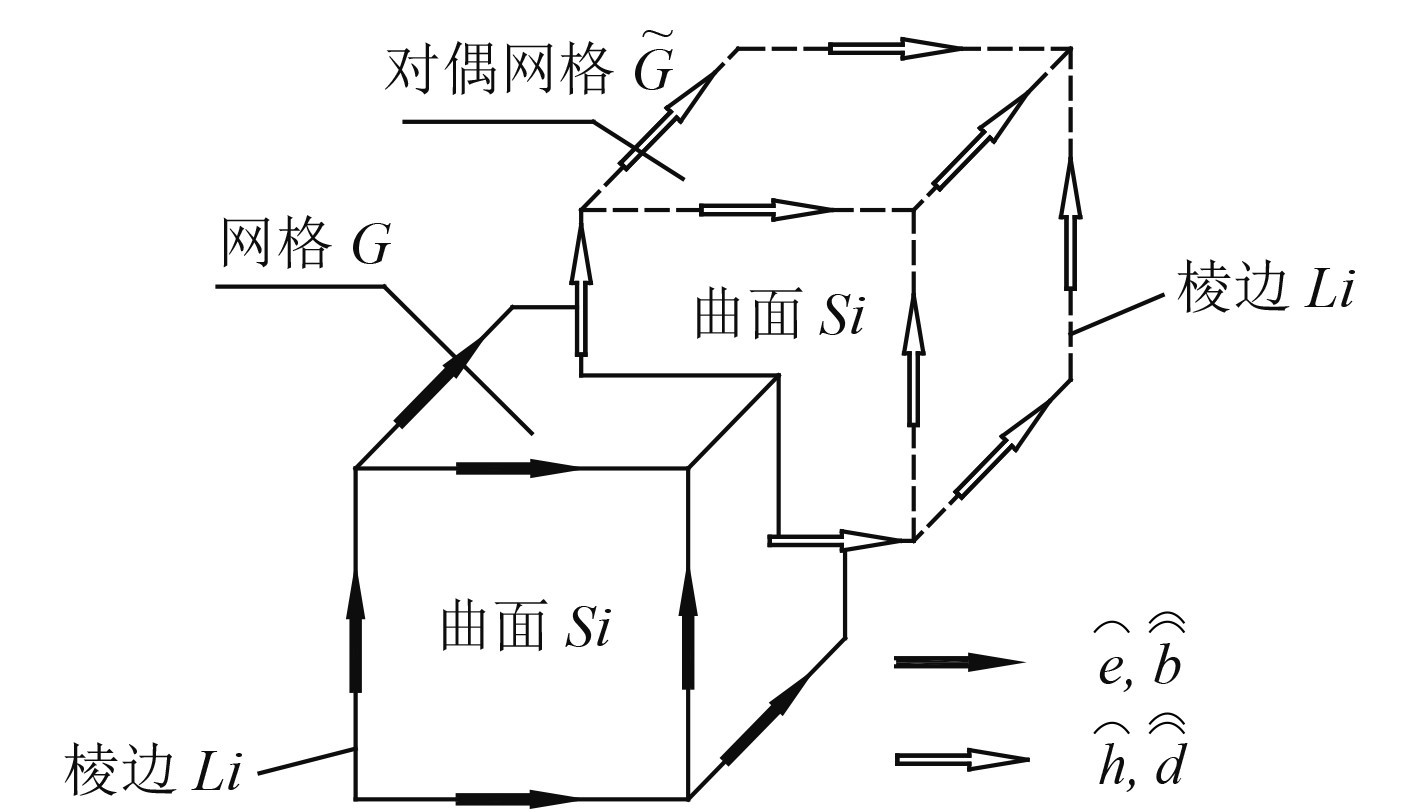图 1 网格G和对偶网格 $\widetilde G$ 的空间关系 Fig. 1 Spatial relationship between grid G and dual grid $\widetilde G$

 $C{\overset{\frown} e} = - \frac{\rm d}{{{\rm d}t}}{\overset\frown{\overset\frown{b}}} {\text{，}}$ (8)
 $\tilde C{\overset{\frown} h} = {\overset\frown{\overset\frown{j}}} + \frac{\rm d}{{{\rm d}t}}{\overset\frown{\overset\frown{d}}}{\text{，}}$ (9)
 $S{\overset\frown{\overset\frown{b}}} = 0{\text{，}}$ (10)
 $\tilde S{\overset\frown{\overset\frown{d}}} = q{\text{。}}$ (11)

 ${\overset\frown{\overset\frown{d}}} = {{{M_\varepsilon}} }{\overset{\frown} e}{\text{，}}$ (12)
 ${\overset\frown{\overset\frown{b}}} = {{{M_\mu}} }{\overset{\frown} h}{\text{，}}$ (13)
 ${\overset\frown{\overset\frown{j}}} = {{{M_\sigma}} }{\overset{\frown} e}{\text{。}}$ (14)

1.3 麦克斯韦方程时域离散求解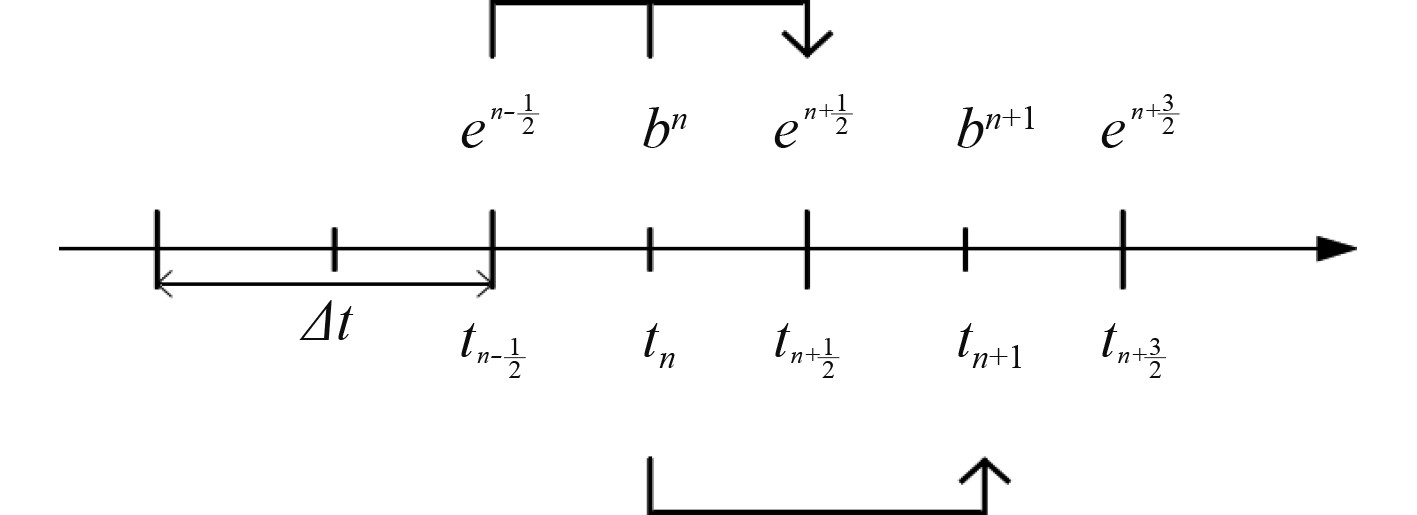图 2 蛙跃跳步法图示 Fig. 2 Picture of leaping frog

 ${{\overset{\frown} e} ^{n + 1/2}} = {{\overset{\frown} e} ^{n - 1/2}} + \Delta t{{M_\varepsilon}} ^{ - 1}\left[{ {\tilde C{{M_\mu}} ^{ - 1}{{{\overset\frown{\overset\frown{b}}}} }^n} + {\overset\frown{\overset\frown{j}}} _S^n} \right]{\text{，}}$ (15)
 ${{\overset\frown{\overset\frown{b}}} ^{n + 1}} = {{\overset\frown{\overset\frown{b}}} ^n} - \Delta tC{{\overset{\frown} e} ^{n + 1/2}}{\text{。}}$ (16)

 $\Delta t \leqslant \frac{{\sqrt {\varepsilon \mu } }}{{\sqrt {\left[ {\frac{1}{{\Delta {x^2}}} + \frac{1}{{\Delta {y^2}}} + \frac{1}{{\Delta {z^2}}}} \right]} }}{\text{。}}$ (17)

2 RCS仿真计算模型 2.1 对象模型参考

2.2 几何建模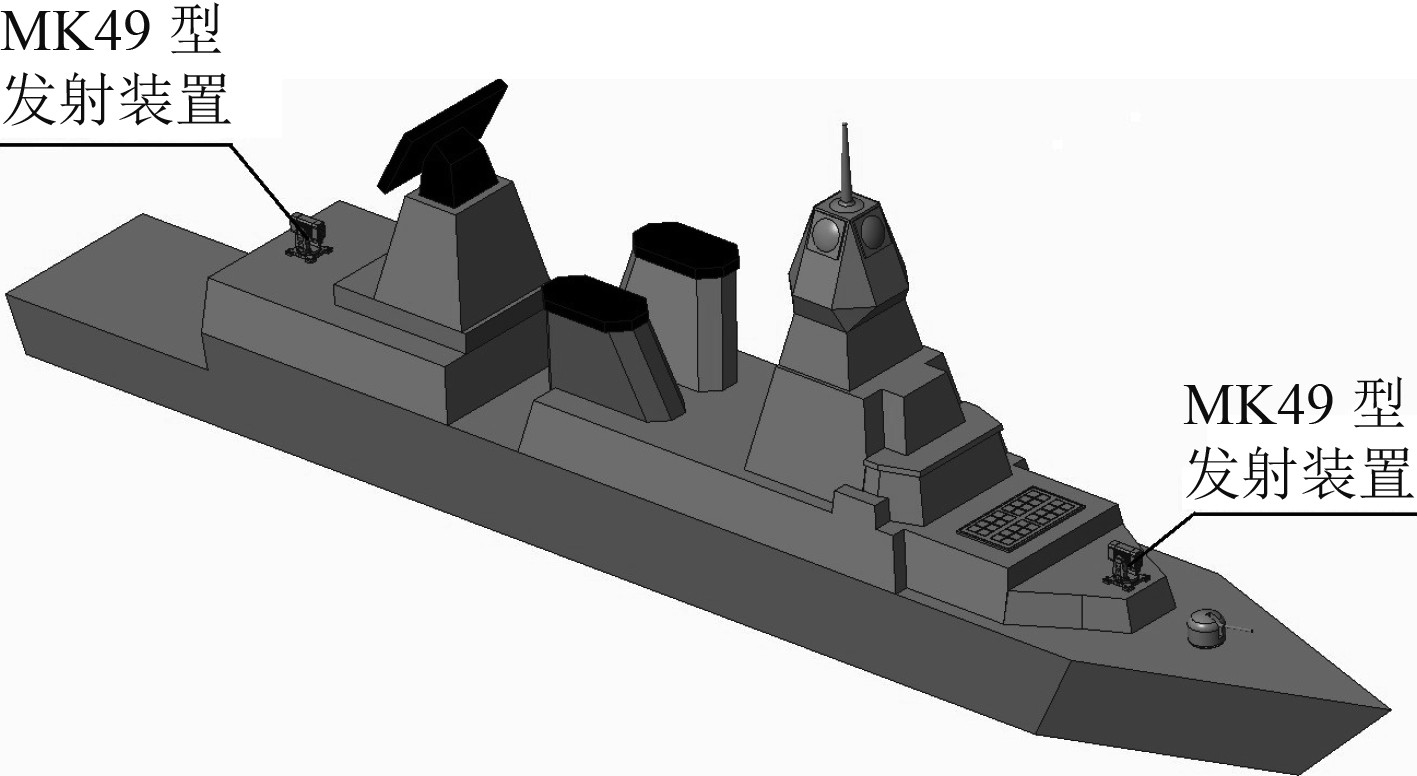图 3 舰船几何模型全貌布置 Fig. 3 Full-view arrangement of ship geometric model
2.3 RCS仿真对象选取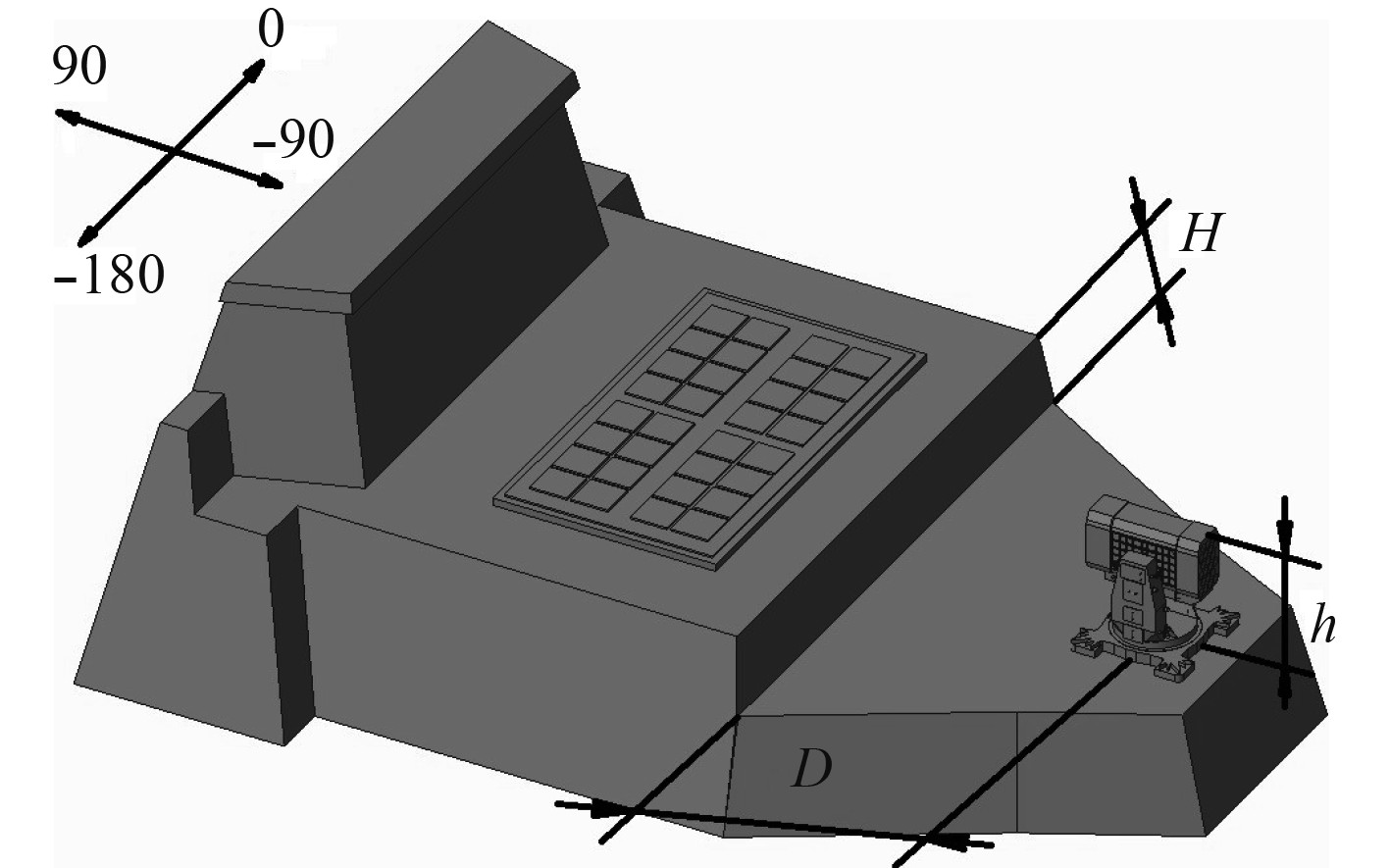图 4 RCS仿真模型计算坐标系 Fig. 4 RCS simulation model calculation coordinate system

3 仿真计算 3.1 耦合特性定义

 ${{RCS}}_{\text{耦合}}={{RCS}}_{\text{舰船}}-({{RCS}}_{\text{平台}}+{{RCS}}_{\text{发射装置}})\text{。}$ (18)

3.2 分析软件及参数设定

3.3 求解计算及结果分析

3.3.1 H为定量、D为变量条件下的求解及分析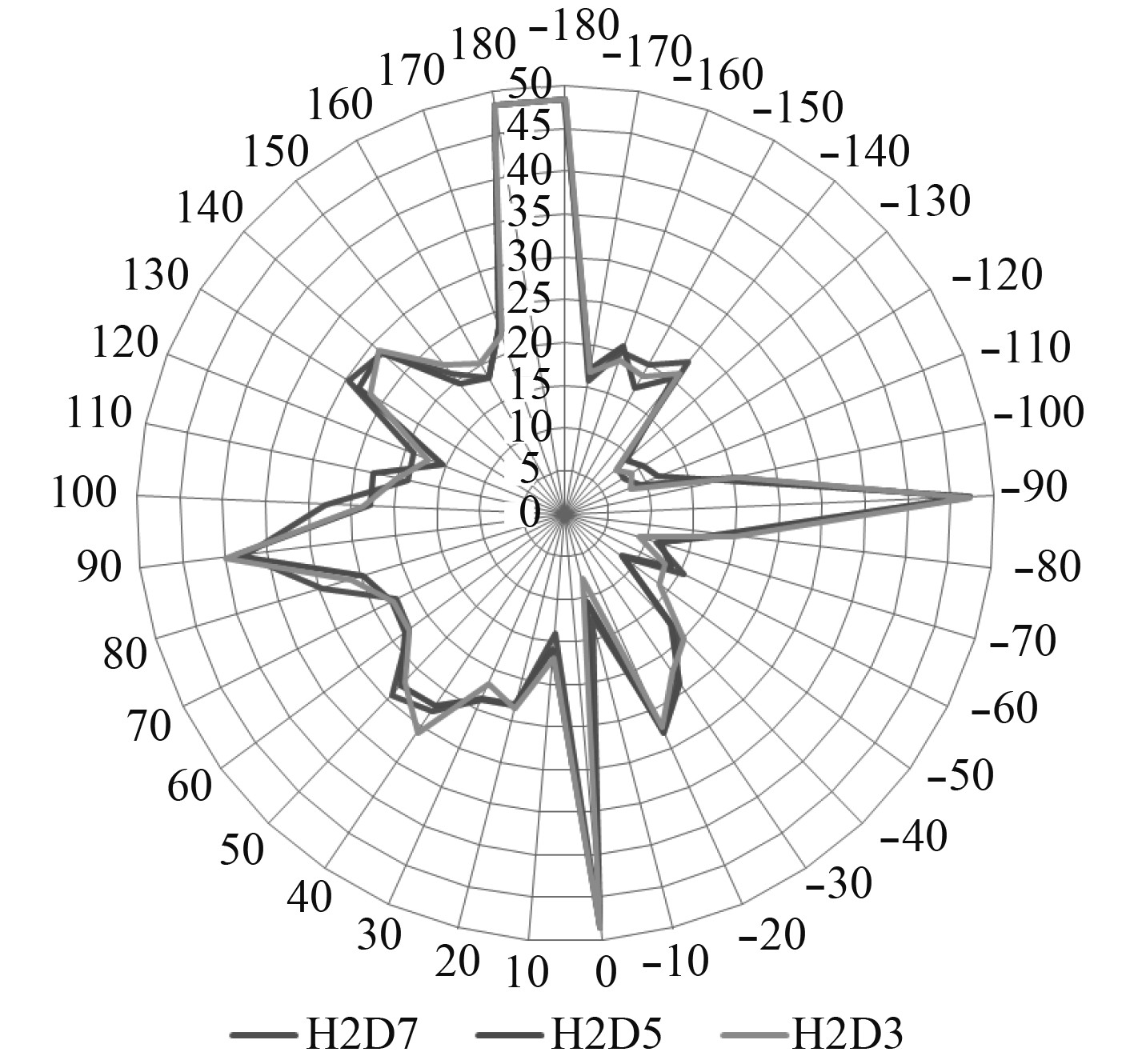图 5 10 GHz条件下RCS舰船计算结果对比 Fig. 5 Comparison of RCSship calculations under 10 GHz condition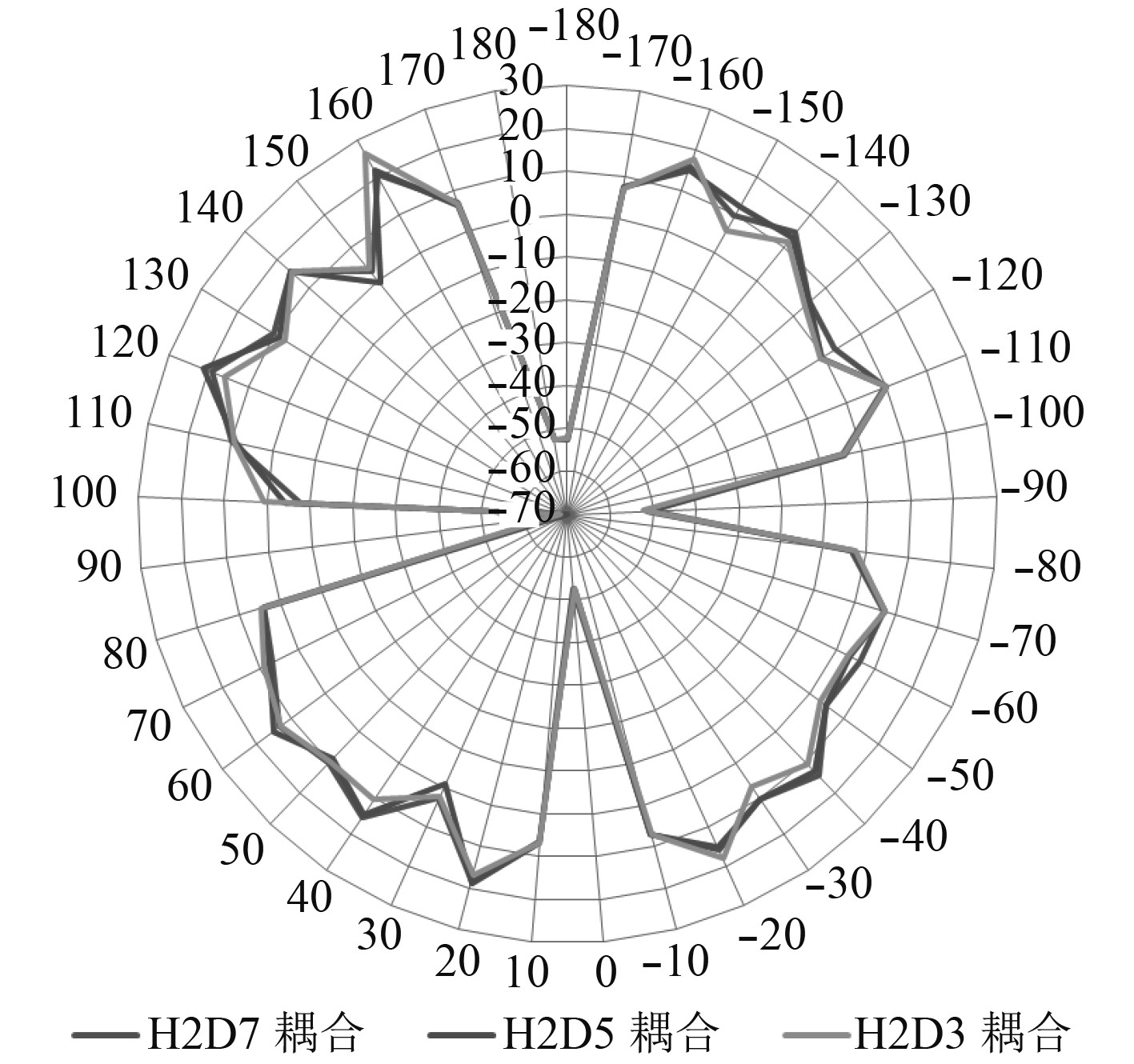图 8 15 GHz条件下RCS耦合计算结果对比 Fig. 8 Comparison of RCScoupling calculations under 15 GHz condition表 1 发射装置距舰船上层建筑不同距离条件下RCS计算结果(H=2) Tab.1 RCS calculation results of launcher at different distances from superstructure of ship(H=2)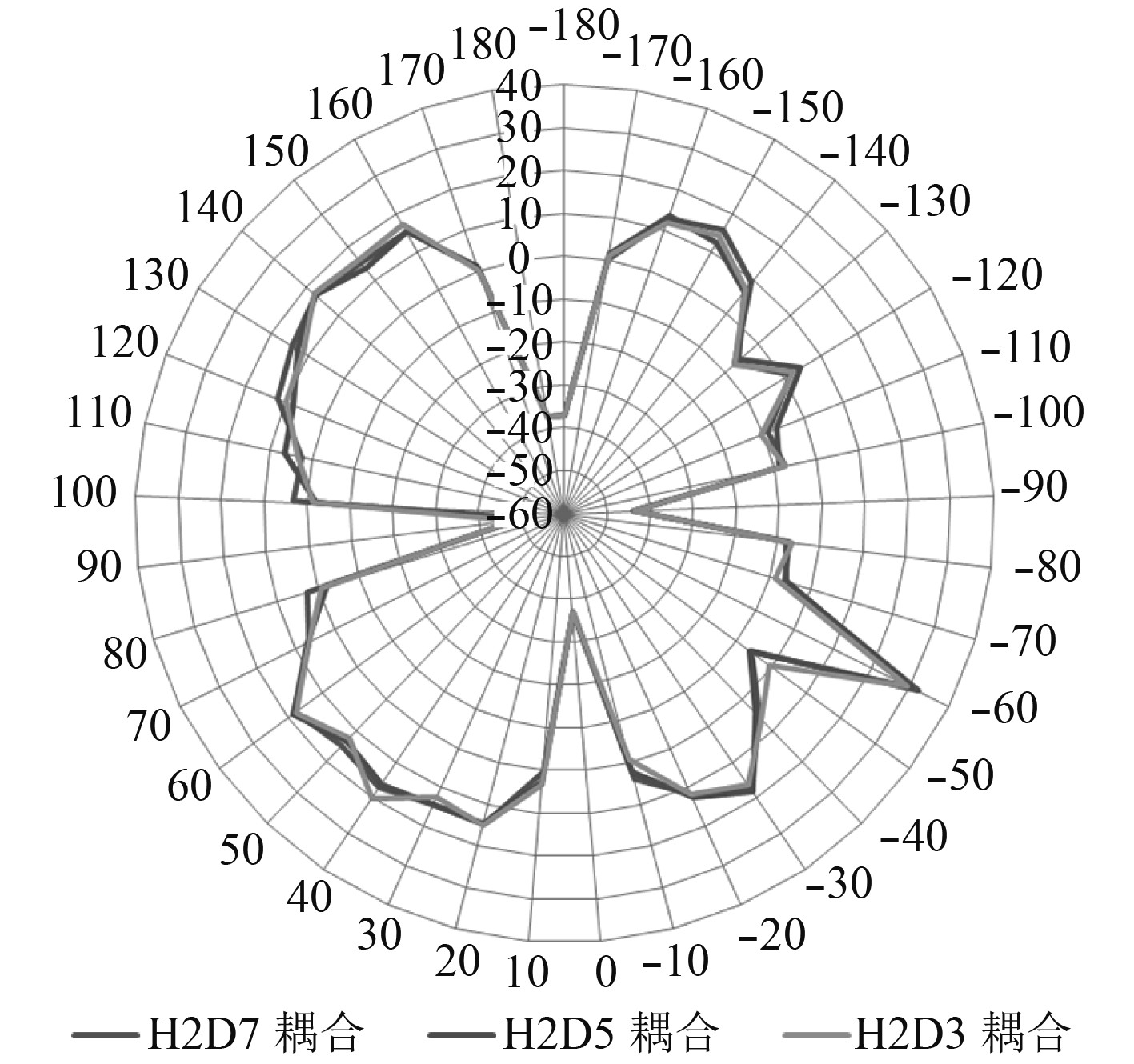图 6 10 GHz条件下RCS耦合计算结果对比 Fig. 6 Comparison of RCScoupling calculations under 10 GHz condition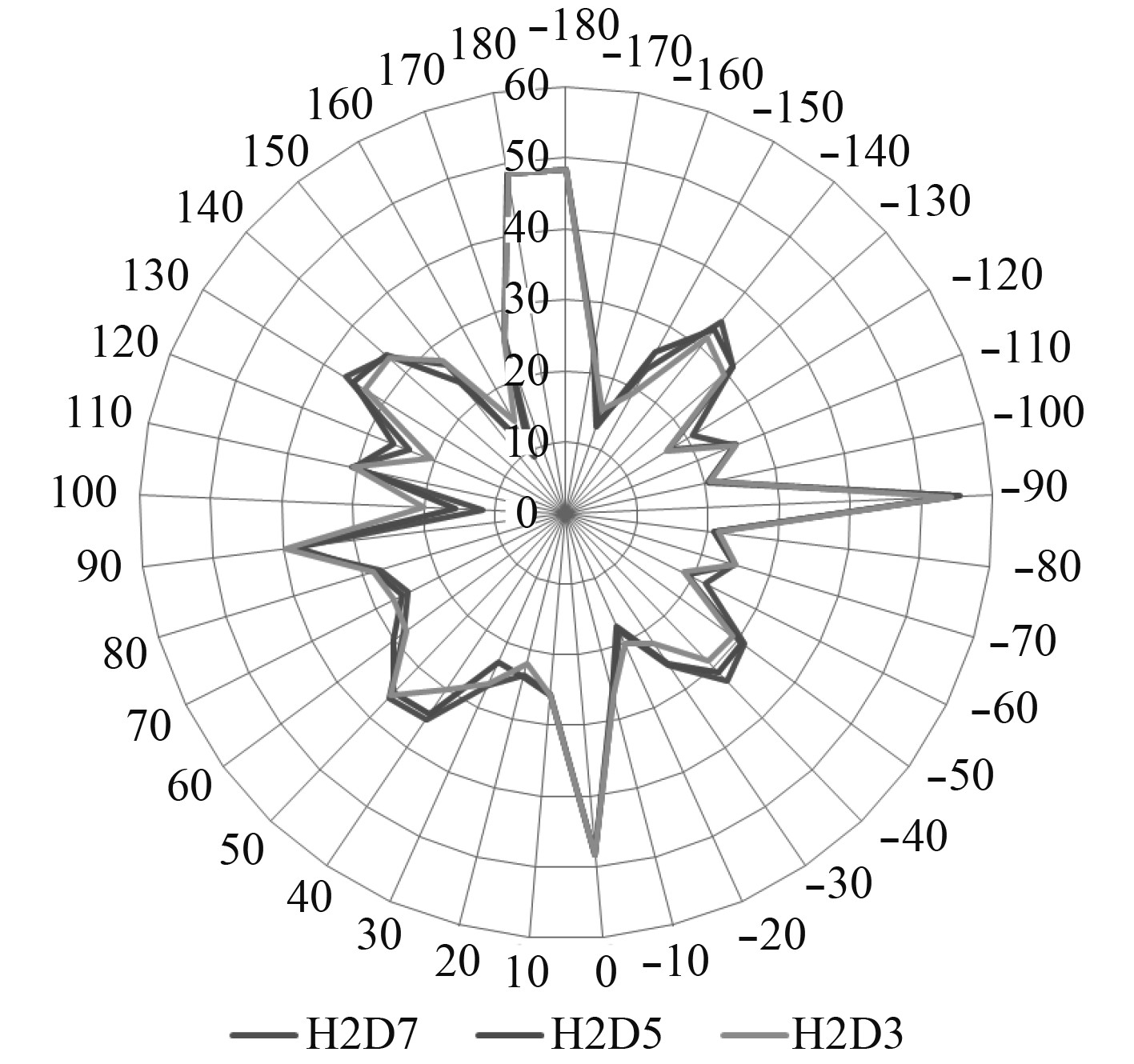图 7 15 GHz条件下RCS舰船计算结果对比 Fig. 7 Comparison of RCSship calculations under 15 GHz condition

3.3.2 D为定量、H为变量条件下的求解及分析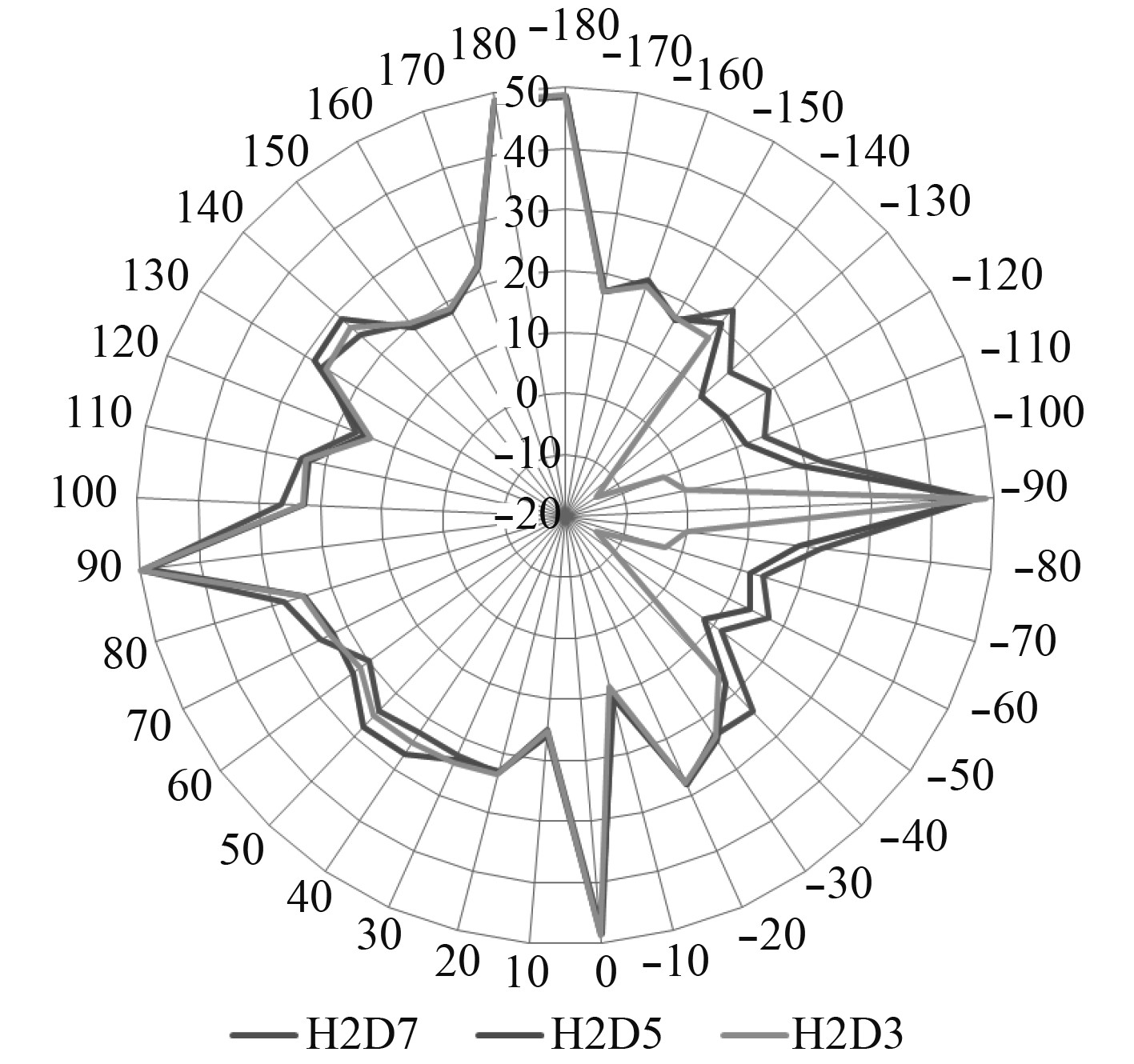图 9 10 GHz条件下RCS舰船计算结果对比 Fig. 9 Comparison of RCSship calculations under 10 GHz condition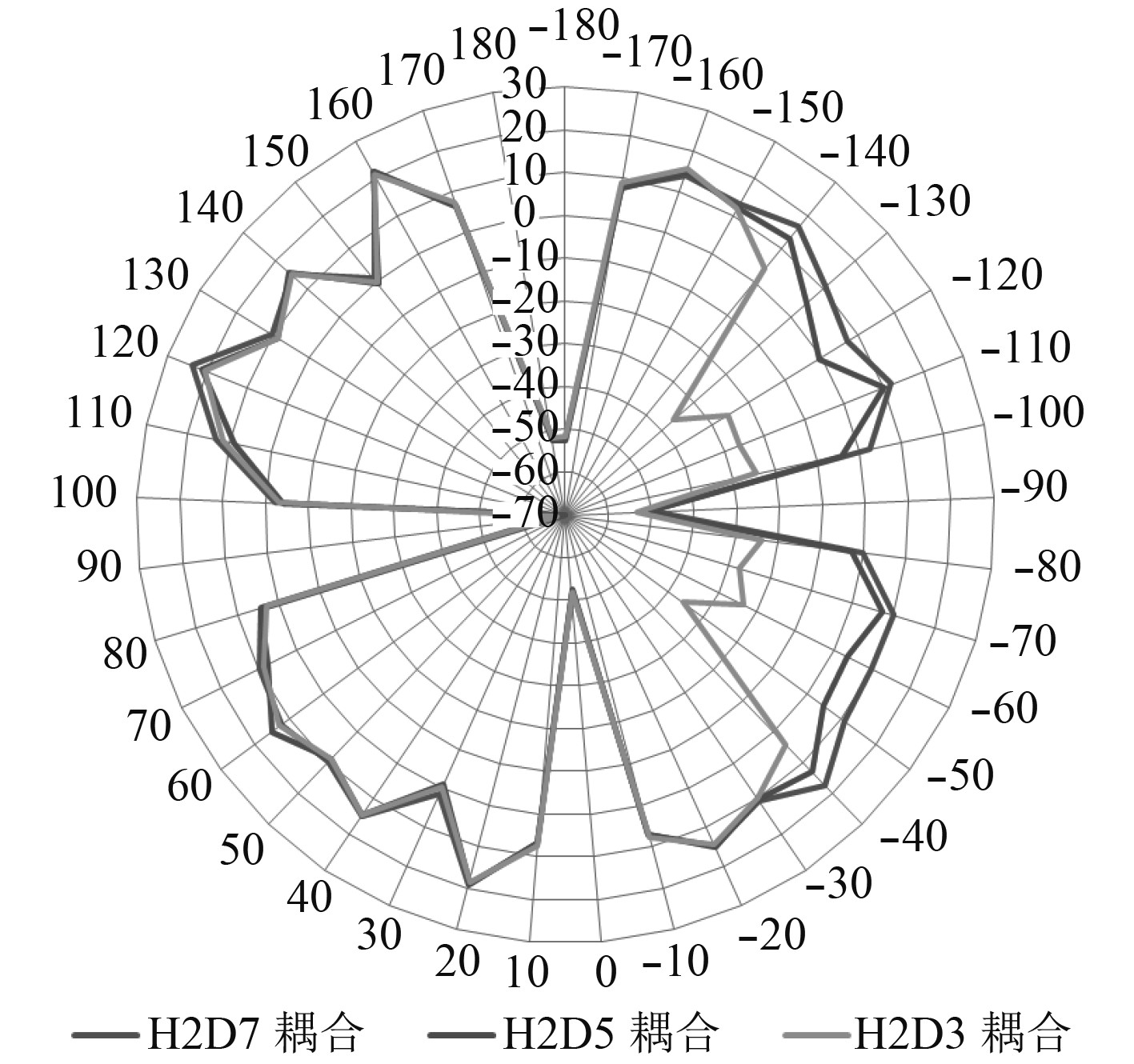图 12 15 GHz条件下RCS耦合计算结果对比 Fig. 12 Comparison of RCScoupling calculations under 15 GHz condition表 2 发射装置距舰船上层建筑不同高度条件下RCS计算结果（D=5） Tab.2 RCS calculation results of launcher at different height from superstructure of ship（D=5）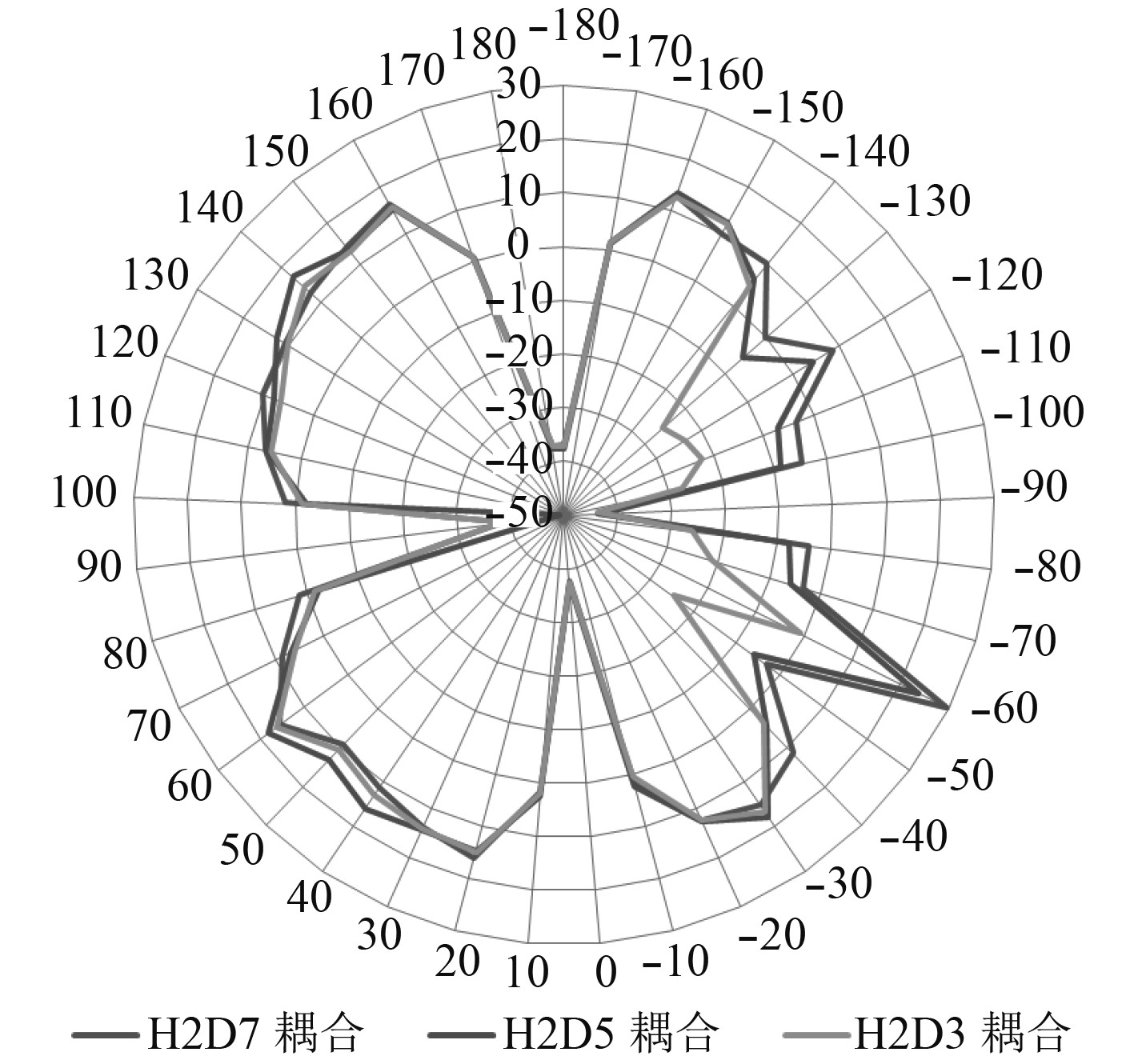图 10 10 GHz条件下RCS耦合计算结果对比 Fig. 10 Comparison of RCScoupling calculations under 10 GHz condition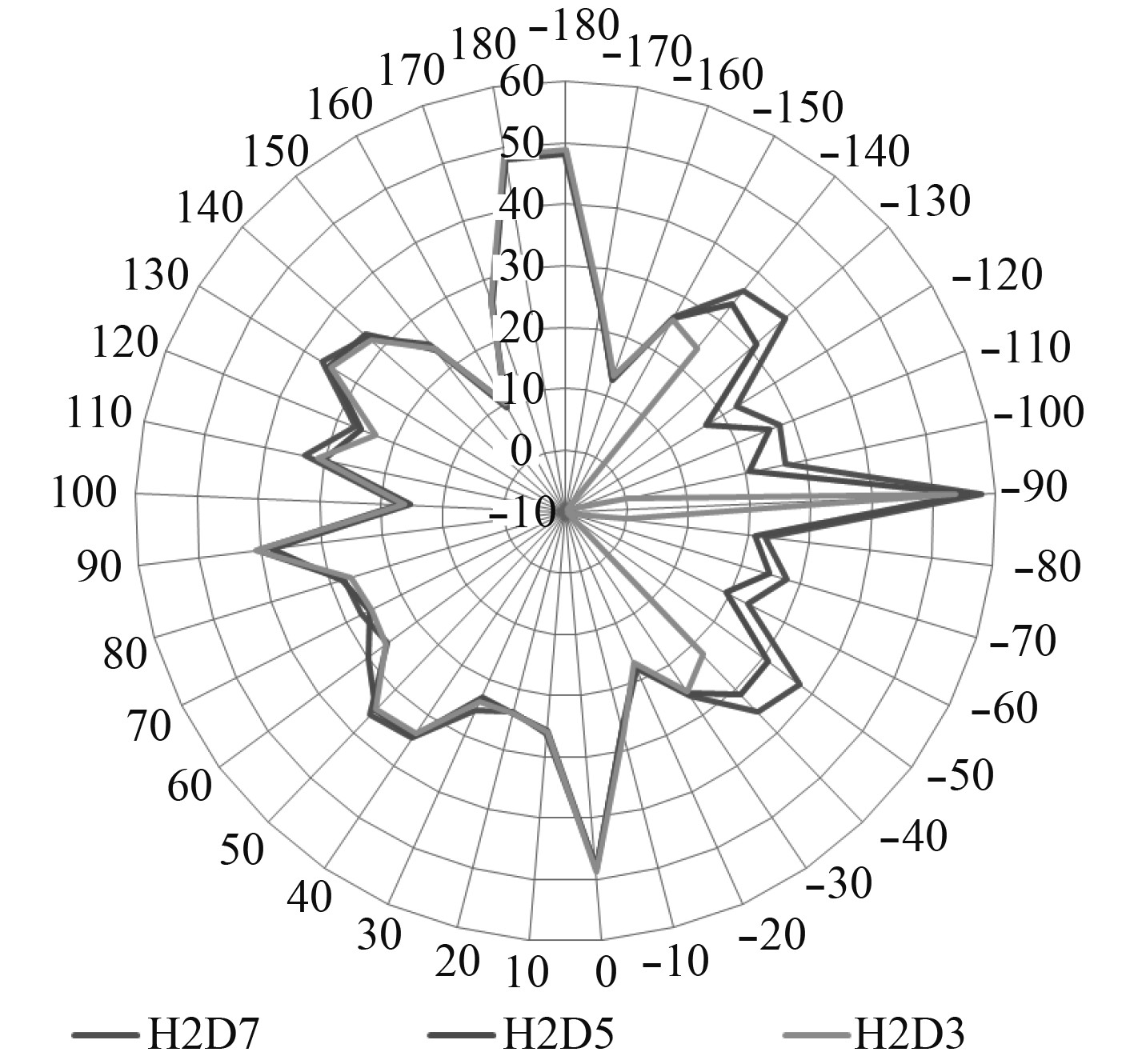图 11 15 GHz条件下RCS舰船计算结果对比 Fig. 11 Comparison of RCSship calculations under 15 GHz condition

4 提高舰船隐身性能的方法 4.1 提高发射装置和舰船上层建筑隐身性能

4.2 改善发射装置与舰船上层建筑的耦合效果

5 结　语

  庄钊文, 袁乃昌, 莫锦军, 等.军用目标雷达散射截面预估与测量 [M]. 北京: 科学出版社, 2007: 1-3.  王伟锋. 阵列天线结构变形与RCS耦合建模及综合性能分析 [D]. 西安: 西安电子科技大学, 2014.  朱英富, 张国良. 舰船隐身技术 [M]. 哈尔滨: 哈尔滨工程大学出版社, 2003: 8-10.  李明智, 熊章强, 张大洲. 有限积分法与有限差分法在弹性波数值模拟中的对比分析[J]. 中国科技论文, 2015, 10(3): 538-541. LI Ming-zhi, XIONG Zhang-qiang, ZHANG Da-zhou. Comparison of elastodynamic finite integration technique and finite difference method in numerical simulation of elastic wave[J]. China Sciencepaper, 2015, 10(3): 538-541.  杨兴宝, 金钊, 朱传伟. 拉姆导弹武器系统近期发展及关键技术分析[J]. 飞航导弹, 2016(7): 25-29.  刘鸿雁, 郑守铎. 反舰导弹标准靶标RCS仿真[J]. 海军航空工程学院学报, 2011, 26(1): 89-92. DOI:10.3969/j.issn.1673-1522.2011.01.021  程子君, 吴启华. 隐身桅杆与主船体耦合RCS特性研究[J]. 中国舰船研究, 2011, 6(3): 45-48, 54. CHENG Zi-jun, WU Qi-hua. The RCS characteristics of coupling between stealth mast and main hull[J]. Chinese Journal of Ship Research, 2011, 6(3): 45-48, 54. DOI:10.3969/j.issn.1673-3185.2011.03.010  宋广, 张德保, 李鸣. 水面舰船RCS统计模型分析[J]. 舰船电子对抗, 2011, 34(2): 73-74, 78. SONG Guang, ZHANG De-bao, LI Ming. Analysis of statistical model for RCS of surface ship[J]. Shipboard Electronic Countermeasure, 2011, 34(2): 73-74, 78. DOI:10.3969/j.issn.1673-9167.2011.02.019